# LeNet Trained onMNIST Data

Identify the handwritten digit in an image

This pioneer work for image classification with convolutional neural nets was released in 1998. It was developed by Yann LeCun and his collaborators at AT&T Labs while they experimented with a large range of machine learning solutions for classification on the MNIST dataset.

Number of layers: 147 | Parameter count: 5,978,677 | Trained size: 1,750 KB |

## Training Set Information

• MNIST Database of Handwritten Digits, consisting of 60,000 training and 10,000 test grayscale images of size 28x28.

## Performance

• This model achieves 98.5% accuracy on the MNIST dataset.

## Examples

### Resource retrieval

Retrieve the pre-trained net:

 In:=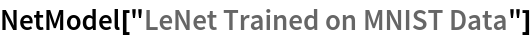Out=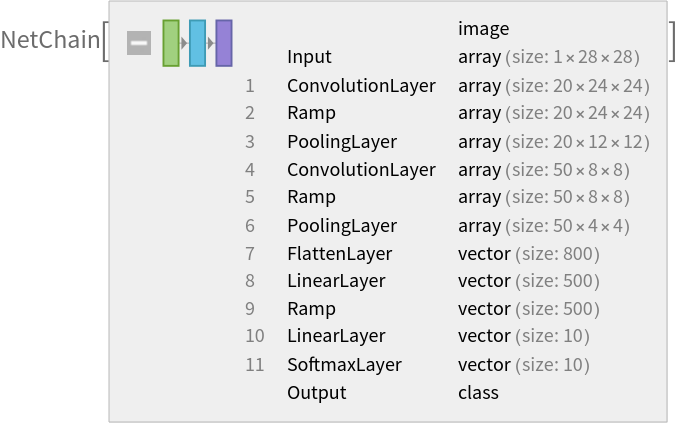### Basic usage

Apply the trained net to a set of inputs:

 In:=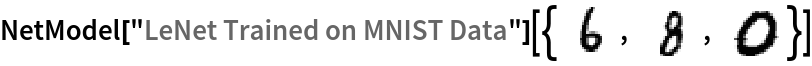Out=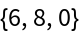Give class probabilities for a single input:

 In:=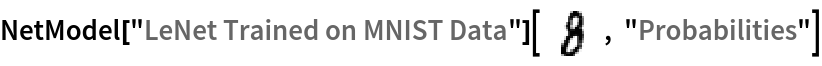Out=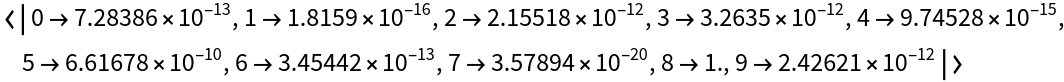### Feature extraction

Create a subset of the MNIST dataset:

 In:=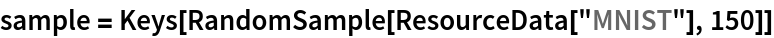Out=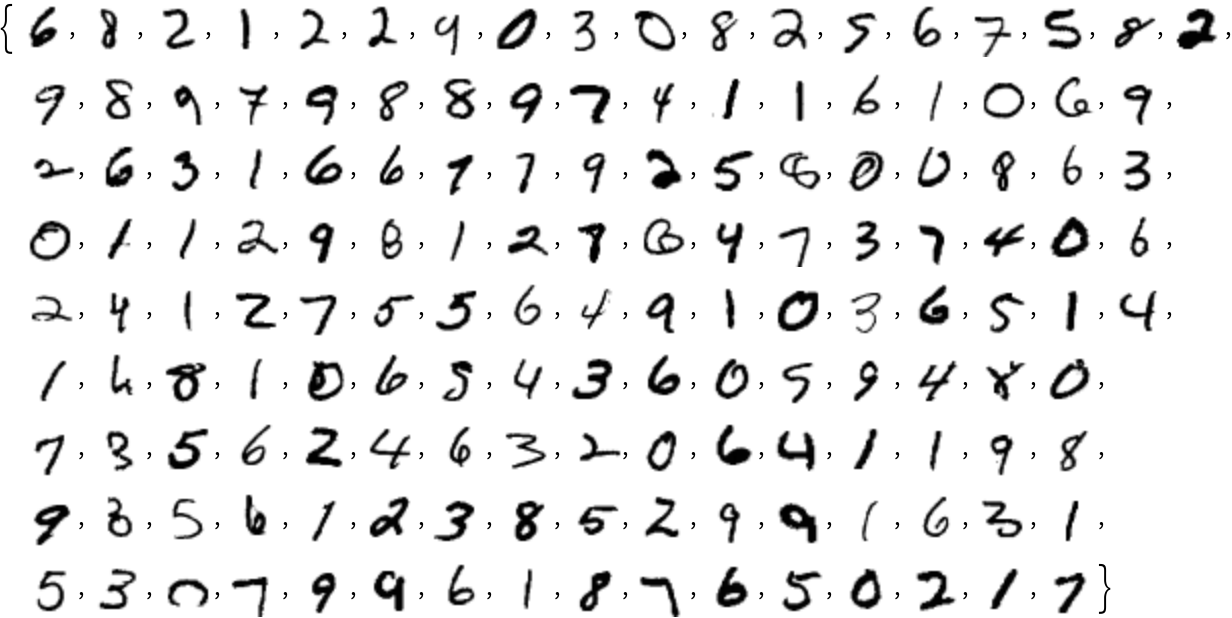Remove the last linear layer of the net, which will be used as a feature extractor:

 In:=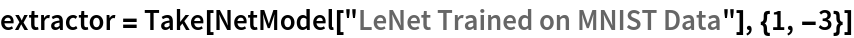Out=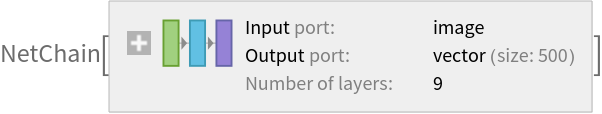Visualize the features of a subset of the MNIST dataset:

 In:=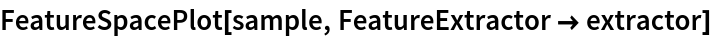Out=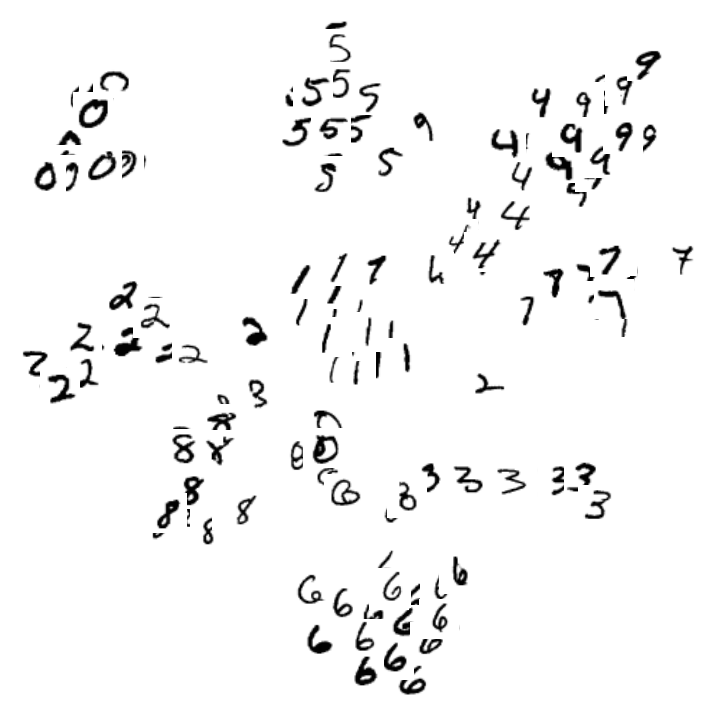### Visualization of net operation

Extract the convolutional features from the first layer:

 In:=Out=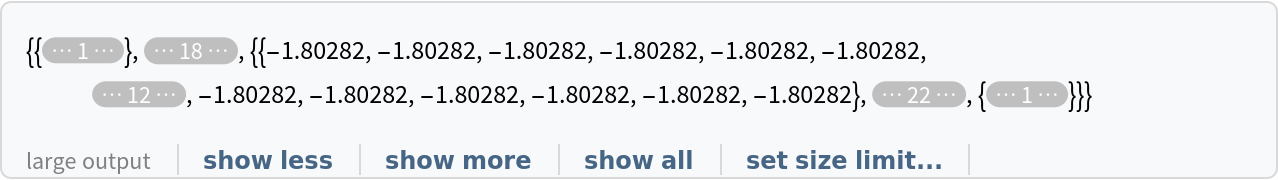Visualize the features:

 In:=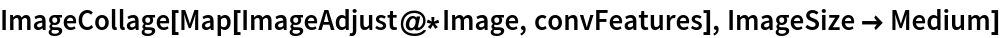Out=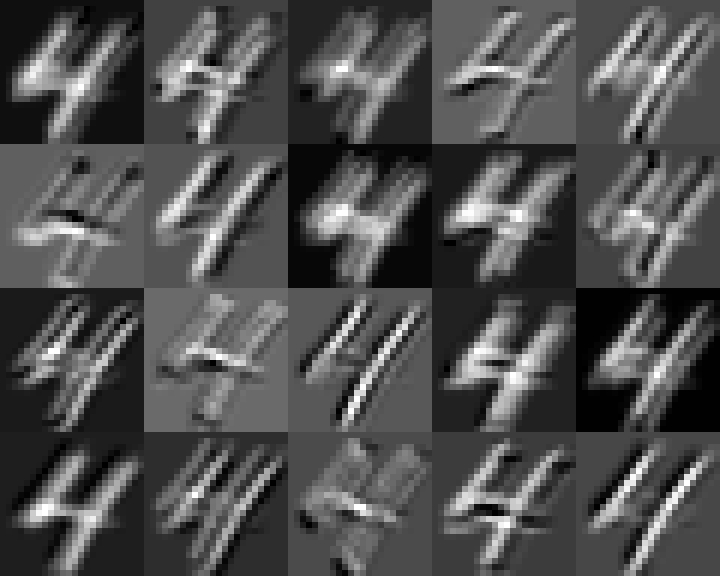### Training the uninitialized architecture

Retrieve the uninitialized architecture:

 In:=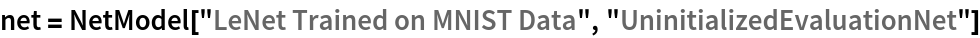Out=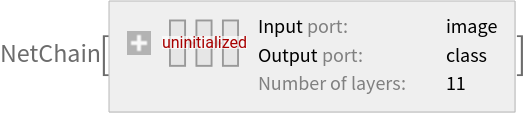Retrieve the MNIST dataset:

 In:=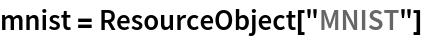Out=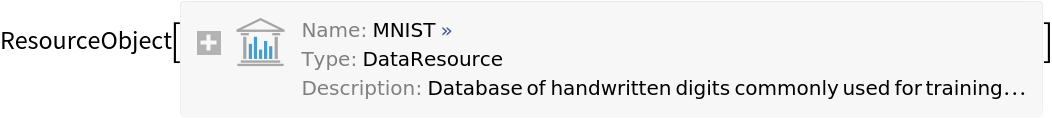Use the training dataset provided:

 In:=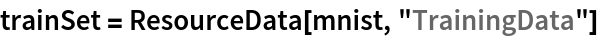Out=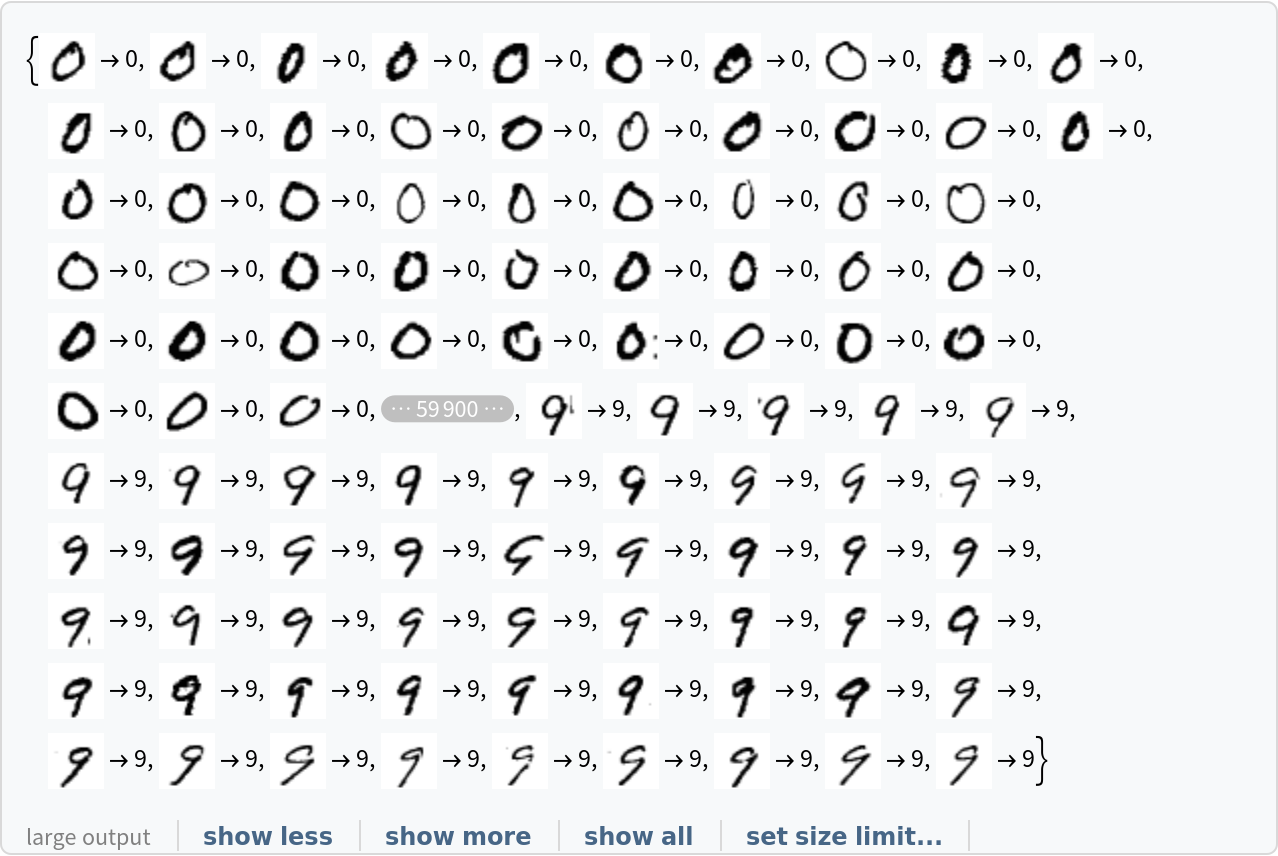Use the test dataset provided:

 In:=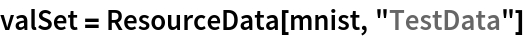Out=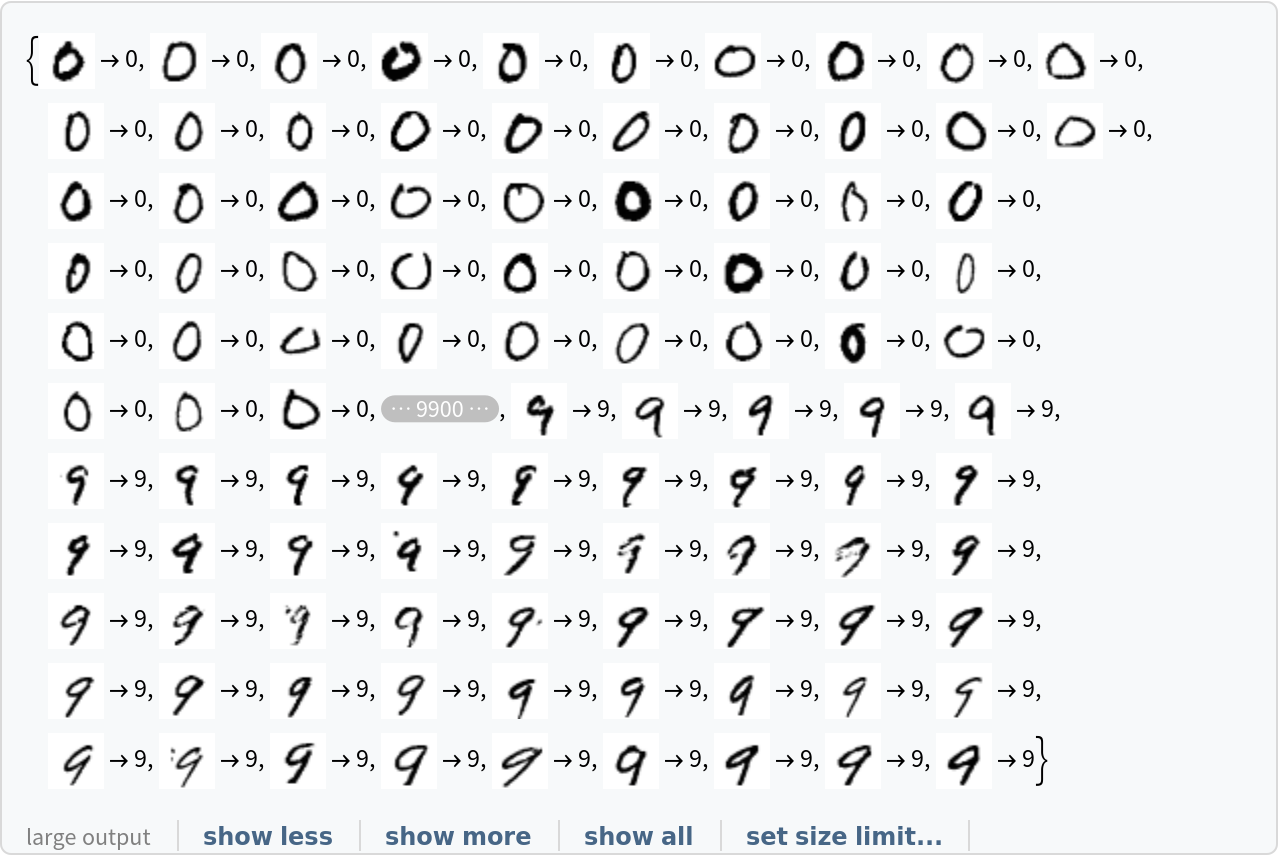Train the net:

 In:=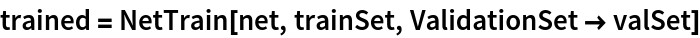Out=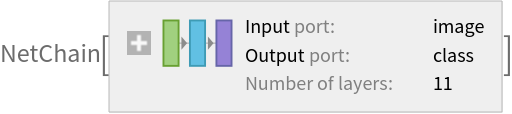Generate a ClassifierMeasurementsObject of the net with the test set:

 In:=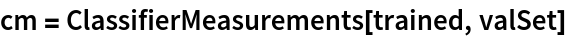Out=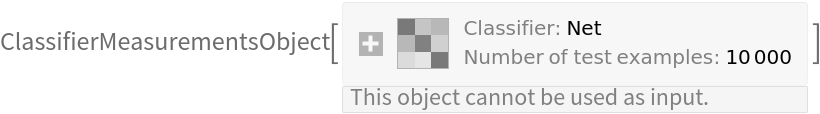Evaluate the accuracy on the validation set:

 In:=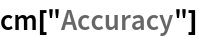Out=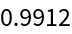Visualize the confusion matrix:

 In:=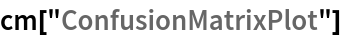Out=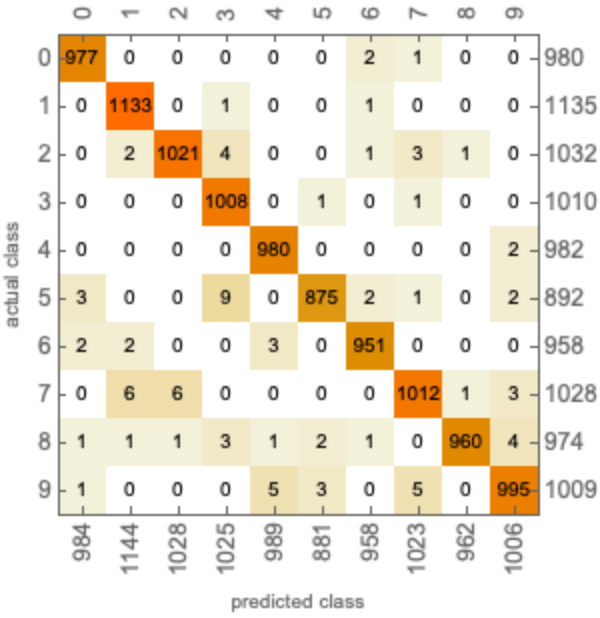### Net information

Inspect the number of parameters of all arrays in the net:

 In:=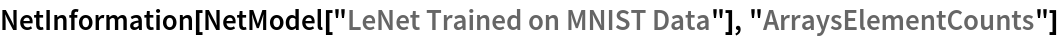Out=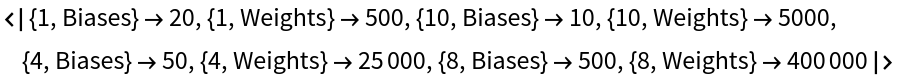Obtain the total number of parameters:

 In:=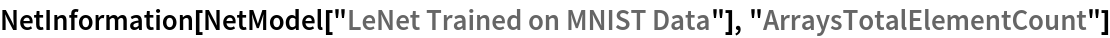Out=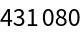Obtain the layer type counts:

 In:=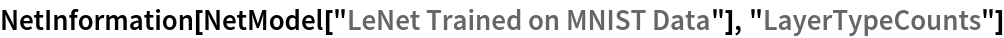Out=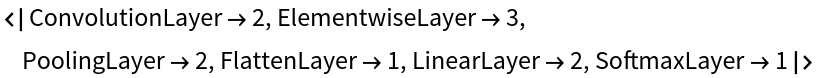Display the summary graphic:

 In:=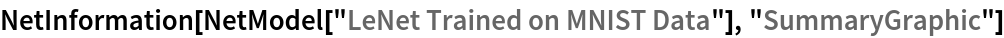Out=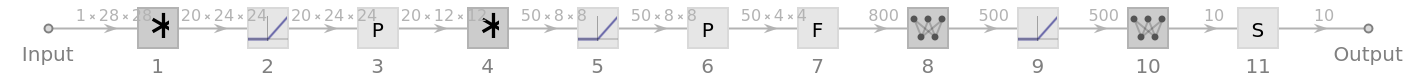### Export to MXNet

Export the net into a format that can be opened in MXNet:

 In:=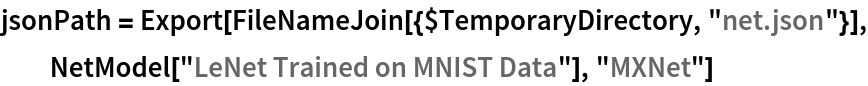Out=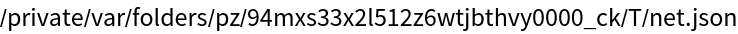Export also creates a net.params file containing parameters:

 In:=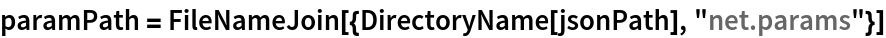Out=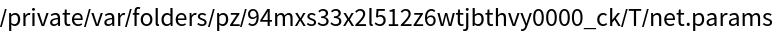Get the size of the parameter file:

 In:=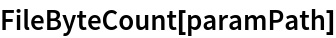Out=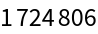The size is similar to the byte count of the resource object:

 In:=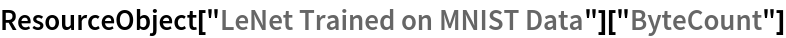Out=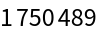Represent the MXNet net as a graph:

 In:=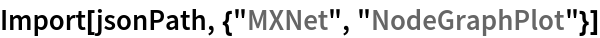Out=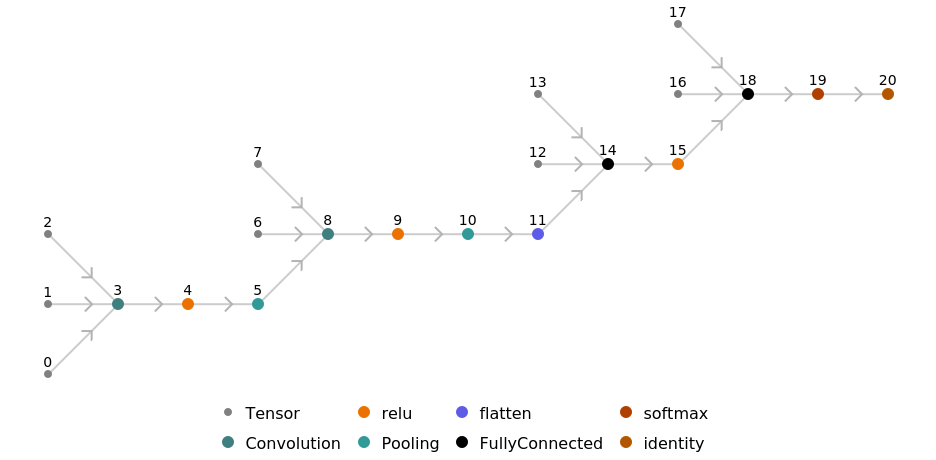## Requirements

Wolfram Language 11.1 (March 2017) or above

## Reference

• Y. LeCun, L. Bottou, Y. Bengio, P. Haffner, "Gradient-Based Learning Applied to Document Recognition," Proceedings of the IEEE, 86(11), 2278-2324 (1998)
• (available from http://yann.lecun.com/exdb/lenet)